# fsurf

Plot 3-D surface

## Syntax

fsurf(f)
fsurf(f,[min max])
fsurf(f,[xmin xmax ymin ymax])
fsurf(funx,funy,funz)
fsurf(funx,funy,funz,[uvmin uvmax])
fsurf(funx,funy,funz,[umin umax vmin vmax])
fsurf(___,LineSpec)
fsurf(___,Name,Value)
fsurf(ax,___)
fs = fsurf(___)

## Description

example

fsurf(f) creates a surface plot of the symbolic expression f(x,y) over the default interval [-5 5] for x and y.

example

fsurf(f,[min max]) plots f(x,y) over the interval [min max] for x and y.

example

fsurf(f,[xmin xmax ymin ymax]) plots f(x,y) over the interval [xmin xmax] for x and [ymin ymax] for y. The fsurf function uses symvar to order the variables and assign intervals.

example

fsurf(funx,funy,funz) plots the parametric surface x = x(u,v), y = y(u,v), z = z(u,v) over the interval [-5 5] for u and v.
fsurf(funx,funy,funz,[uvmin uvmax]) plots the parametric surface x = x(u,v), y = y(u,v), z = z(u,v) over the interval [uvmin uvmax] for u and v.

example

fsurf(funx,funy,funz,[umin umax vmin vmax]) plots the parametric surface x = x(u,v), y = y(u,v), z = z(u,v) over the interval [umin umax] for u and [vmin vmax] for v. The fsurf function uses symvar to order the parametric variables and assign intervals.

example

fsurf(___,LineSpec) uses LineSpec to set the line style, marker symbol, and face color. Use this option after any of the previous input argument combinations.

example

fsurf(___,Name,Value) specifies line properties using one or more Name,Value pair arguments. Use this option after any of the input argument combinations in the previous syntaxes.
fsurf(ax,___) plots into the axes with the object ax instead of the current axes object gca.

example

fs = fsurf(___) returns a function surface object or parameterized function surface object, depending on the type of surface. Use the object to query and modify properties of a specific surface. For details, see FunctionSurface Properties and ParameterizedFunctionSurface Properties.

## Examples

### 3-D Surface Plot of Symbolic Expression

Plot the input $\mathrm{sin}\left(x\right)+\mathrm{cos}\left(y\right)$ over the default range $-5 and $-5.

syms x y fsurf(sin(x)+cos(y))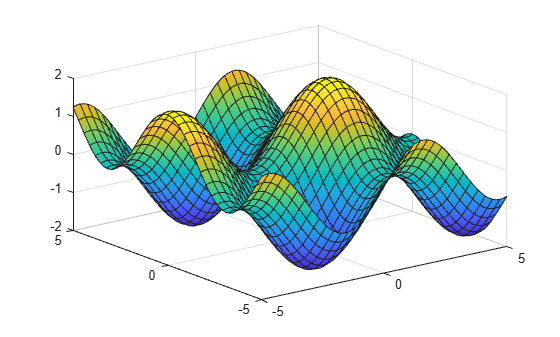### 3-D Surface Plot of Symbolic Function

Plot the real part of ${tan}^{-1}\left(x+iy\right)$ over the default range $-5 and $-5.

syms f(x,y) f(x,y) = real(atan(x + i*y)); fsurf(f)### Specify Plotting Interval of Surface Plot

Plot $\mathrm{sin}\left(x\right)+\mathrm{cos}\left(y\right)$ over $-\pi and $-5 by specifying the plotting interval as the second argument of fsurf.

syms x y f = sin(x) + cos(y); fsurf(f, [-pi pi -5 5])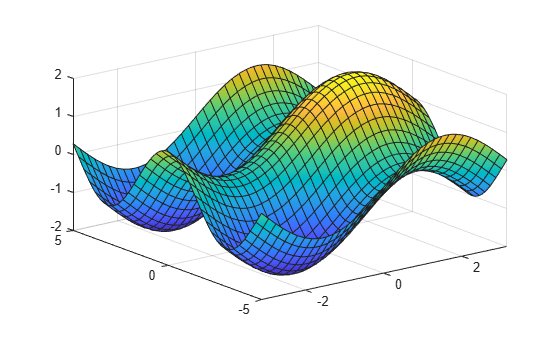### Parameterized Surface Plot

Plot the parameterized surface

$\begin{array}{c}x=r\mathrm{cos}\left(s\right)\mathrm{sin}\left(t\right)\\ y=r\mathrm{sin}\left(s\right)\mathrm{sin}\left(t\right)\\ z=r\mathrm{cos}\left(t\right)\\ where\phantom{\rule{1em}{0ex}}r=2+\mathrm{sin}\left(7s+5t\right)\end{array}$

for $0 and $0.

Improve the plot's appearance by using camlight.

syms s t r = 2 + sin(7*s + 5*t); x = r*cos(s)*sin(t); y = r*sin(s)*sin(t); z = r*cos(t); fsurf(x, y, z, [0 2*pi 0 pi]) camlight view(46,52)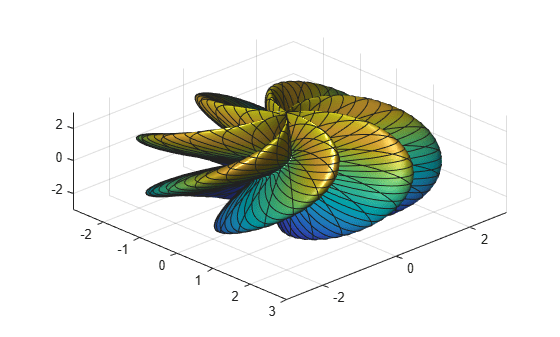### Surface Plot of Piecewise Expression

Plot the piecewise expression of the Klein bottle

$\begin{array}{l}\mathit{x}\left(\mathit{u},\mathit{v}\right)=\left\{\begin{array}{cc}-4\text{\hspace{0.17em}}\mathrm{cos}\left(\mathit{u}\right)\text{\hspace{0.17em}}\left[1+\mathrm{sin}\left(\mathit{u}\right)\right]-\mathit{r}\left(\mathit{u}\right)\text{\hspace{0.17em}}\mathrm{cos}\left(\mathit{u}\right)\text{\hspace{0.17em}}\mathrm{cos}\left(\mathit{v}\right)& 0<\mathit{u}\le \pi \text{\hspace{0.17em}}\\ -4\text{\hspace{0.17em}}\mathrm{cos}\left(\mathit{u}\right)\text{\hspace{0.17em}}\left[1+\mathrm{sin}\left(\mathit{u}\right)\right]+\mathit{r}\left(\mathit{u}\right)\text{\hspace{0.17em}}\mathrm{cos}\left(\mathit{v}\right)& \pi \text{\hspace{0.17em}}<\mathit{u}<\mathrm{2}\pi \text{\hspace{0.17em}}\end{array}\\ \mathit{y}\left(\mathit{u},\mathit{v}\right)=\mathit{r}\left(\mathit{u}\right)\text{\hspace{0.17em}}\mathrm{sin}\left(\mathit{v}\right)\\ \mathit{z}\left(\mathit{u},\mathit{v}\right)=\left\{\begin{array}{cc}-14\text{\hspace{0.17em}}\mathrm{sin}\left(\mathit{u}\right)-\mathit{r}\left(\mathit{u}\right)\text{\hspace{0.17em}}\mathrm{sin}\left(\mathit{u}\right)\text{\hspace{0.17em}}\mathrm{cos}\left(\mathit{v}\right)& 0<\mathit{u}\le \pi \text{\hspace{0.17em}}\\ -14\text{\hspace{0.17em}}\mathrm{sin}\left(\mathit{u}\right)& \pi <\mathit{u}<\mathrm{2}\pi \text{\hspace{0.17em}}\end{array}\\ \mathrm{where}\text{\hspace{0.17em}}\mathit{r}\left(\mathit{u}\right)=4-2\text{\hspace{0.17em}}\mathrm{cos}\left(\mathit{u}\right)\end{array}$

for $0<\mathit{u}<2\pi$ and $0<\mathit{v}<2\pi$.

Show that the Klein bottle has only a one-sided surface.

syms u v; r = @(u) 4 - 2*cos(u); x = piecewise(u <= pi, -4*cos(u)*(1+sin(u)) - r(u)*cos(u)*cos(v),... u > pi, -4*cos(u)*(1+sin(u)) + r(u)*cos(v)); y = r(u)*sin(v); z = piecewise(u <= pi, -14*sin(u) - r(u)*sin(u)*cos(v),... u > pi, -14*sin(u)); h = fsurf(x,y,z, [0 2*pi 0 2*pi]);### Add Title and Axis Labels and Format Ticks

For $x$ and $y$ from $-2\pi$ to $2\pi$, plot the 3-D surface $y\mathrm{sin}\left(x\right)-x\mathrm{cos}\left(y\right)$. Add a title and axis labels.

Create the x-axis ticks by spanning the x-axis limits at intervals of pi/2. Convert the axis limits to precise multiples of pi/2 by using round and get the symbolic tick values in S. Display these ticks by using the XTick property. Create x-axis labels by using arrayfun to apply texlabel to S. Display these labels by using the XTickLabel property. Repeat these steps for the y-axis.

To use LaTeX in plots, see latex.

syms x y fsurf(y.*sin(x)-x.*cos(y), [-2*pi 2*pi]) title('ysin(x) - xcos(y) for x and y in [-2\pi,2\pi]') xlabel('x') ylabel('y') zlabel('z') ax = gca; S = sym(ax.XLim(1):pi/2:ax.XLim(2)); S = sym(round(vpa(S/pi*2))*pi/2); ax.XTick = double(S); ax.XTickLabel = arrayfun(@texlabel,S,'UniformOutput',false); S = sym(ax.YLim(1):pi/2:ax.YLim(2)); S = sym(round(vpa(S/pi*2))*pi/2); ax.YTick = double(S); ax.YTickLabel = arrayfun(@texlabel,S,'UniformOutput',false);### Line Style and Width for Surface Plot

Plot the parametric surface $x=s\mathrm{sin}\left(t\right)$, $y=-s\mathrm{cos}\left(t\right)$, $z=t$ with different line styles for different values of $t$. For $-5, use a dashed line with green dot markers. For $-2, use a LineWidth of 1 and a green face color. For $2, turn off the lines by setting EdgeColor to none.

syms s t fsurf(s*sin(t),-s*cos(t),t,[-5 5 -5 -2],'--.','MarkerEdgeColor','g') hold on fsurf(s*sin(t),-s*cos(t),t,[-5 5 -2 2],'LineWidth',1,'FaceColor','g') fsurf(s*sin(t),-s*cos(t),t,[-5 5 2 5],'EdgeColor','none')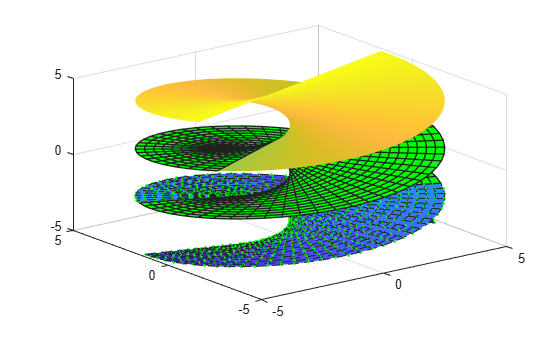### Modify Surface After Creation

Plot the parametric surface

$\begin{array}{c}x={e}^{-|u|/10}\mathrm{sin}\left(5|v|\right)\\ y={e}^{-|u|/10}\mathrm{cos}\left(5|v|\right)\\ z=u.\end{array}$

Specify an output to make fcontour return the plot object.

syms u v x = exp(-abs(u)/10).*sin(5*abs(v)); y = exp(-abs(u)/10).*cos(5*abs(v)); z = u; fs = fsurf(x,y,z)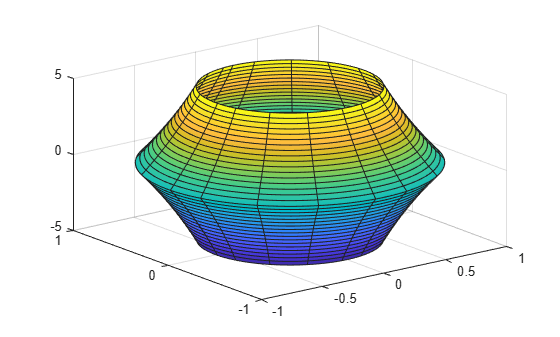fs = ParameterizedFunctionSurface with properties: XFunction: [1x1 sym] YFunction: [1x1 sym] ZFunction: [1x1 sym] EdgeColor: [0 0 0] LineStyle: '-' FaceColor: 'interp' Show all properties 

Change the range of u to [-30 30] by using the URange property of fs. Set the line color to blue by using the EdgeColor property and specify white, dot markers by using the Marker and MarkerEdgeColor properties.

fs.URange = [-30 30]; fs.EdgeColor = 'b'; fs.Marker = '.'; fs.MarkerEdgeColor = 'w';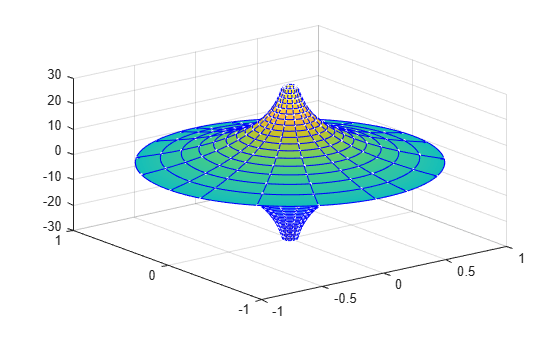### Multiple Surface Plots and Transparent Surfaces

Plot multiple surfaces using vector input to fsurf. Alternatively, use hold on to plot successively on the same figure. When displaying multiple surfaces on the same figure, transparency is useful. Adjust the transparency of surface plots by using the FaceAlpha property. FaceAlpha varies from 0 to 1, where 0 is full transparency and 1 is no transparency.

Plot the planes $x+y$ and $x-y$ using vector input to fsurf. Show both planes by making them half transparent using FaceAlpha.

syms x y h = fsurf([x+y x-y]); h(1).FaceAlpha = 0.5; h(2).FaceAlpha = 0.5; title('Planes (x+y) and (x-y) at half transparency')### Control Resolution of Surface Plot

Control the resolution of a surface plot using the 'MeshDensity' option. Increasing 'MeshDensity' can make smoother, more accurate plots while decreasing it can increase plotting speed.

Divide a figure into two using subplot. In the first subplot, plot the parametric surface $x=\mathrm{sin}\left(s\right)$, $y=\mathrm{cos}\left(s\right)$, and $z=\left(t/10\right)\mathrm{sin}\left(1/s\right)$. The surface has a large gap. Fix this issue by increasing the 'MeshDensity' to 40 in the second subplot. fsurf fills the gap showing that by increasing 'MeshDensity' you increased the plot's resolution.

syms s t subplot(2,1,1) fsurf(sin(s), cos(s), t/10.*sin(1./s)) view(-172,25) title('Default MeshDensity = 35') subplot(2,1,2) fsurf(sin(s), cos(s), t/10.*sin(1./s),'MeshDensity',40) view(-172,25) title('Increased MeshDensity = 40')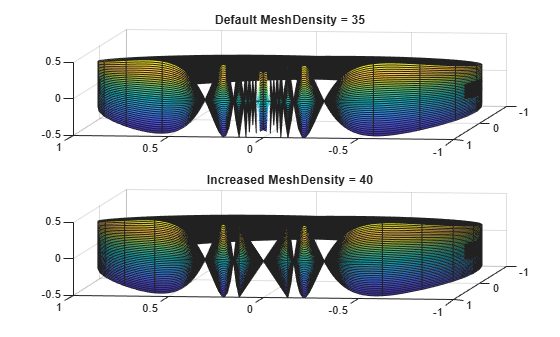### Show Contours Below Surface Plot

Show contours for the surface plot of the expression f by setting the 'ShowContours' option to 'on'.

syms x y f = 3*(1-x)^2*exp(-(x^2)-(y+1)^2)... - 10*(x/5 - x^3 - y^5)*exp(-x^2-y^2)... - 1/3*exp(-(x+1)^2 - y^2); fsurf(f,[-3 3],'ShowContours','on')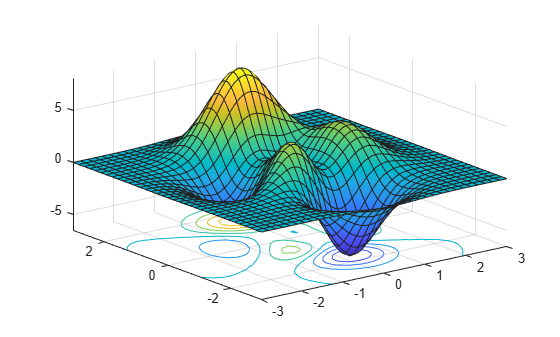### Create Animations of Surface Plots

Create animations by changing the displayed expression using the Function, XFunction, YFunction, and ZFunction properties and then by using drawnow to update the plot. To export to GIF, see imwrite.

By varying the variable i from 1 to 3, animate the parametric surface

$\begin{array}{l}x=t\mathrm{sin}\left(s\right)\\ y=\mathrm{cos}\left(s\right)\\ z=\mathrm{sin}\left(\frac{i}{s}\right).\end{array}$

for -0.1 < u < 0.1 and 0 < v < 1. Increase plotting speed by reducing MeshDensity to 9.

syms s t h = fsurf(t.*sin(s), cos(s), sin(1./s), [-0.1 0.1 0 1]); h.MeshDensity = 9; for i=1:0.05:3 h.ZFunction = sin(i./s); drawnow end### Improve Appearance of Surface Plot

Create a symbolic expression f for the function

$f=3\left(1-x{\right)}^{2}\mathrm{exp}\left(-\left({x}^{2}\right)-\left(y+1{\right)}^{2}\right)-10\left(x/5-{x}^{3}-{y}^{5}\right)\mathrm{exp}\left(-{x}^{2}-{y}^{2}\right)-1/3\mathrm{exp}\left(-\left(x+1{\right)}^{2}-{y}^{2}\right).$

Plot the expression f as a surface. Improve the appearance of the surface plot by using the properties of the handle returned by fsurf, the lighting properties, and the colormap.

Create a light by using camlight. Increase brightness by using brighten. Remove the lines by setting EdgeColor to 'none'. Increase the ambient light using AmbientStrength. For details, see Lighting, Transparency, and Shading (MATLAB). Turn the axes box on. For the title, convert f to LaTeX using latex. Finally, to improve the appearance of the axes ticks, axes labels, and title, set 'Interpreter' to 'latex'.

syms x y f = 3*(1-x)^2*exp(-(x^2)-(y+1)^2)... - 10*(x/5 - x^3 - y^5)*exp(-x^2-y^2)... - 1/3*exp(-(x+1)^2 - y^2); h = fsurf(f,[-3 3]); camlight(110,70) brighten(0.6) h.EdgeColor = 'none'; h.AmbientStrength = 0.4; a = gca; a.TickLabelInterpreter = 'latex'; a.Box = 'on'; a.BoxStyle = 'full'; xlabel('$x$','Interpreter','latex') ylabel('$y$','Interpreter','latex') zlabel('$z$','Interpreter','latex') title_latex = ['$' latex(f) '$']; title(title_latex,'Interpreter','latex')### Surface Plot with Bounded Plane

Plot a cylindrical shell bounded below by the $x-y$ plane and above by the plane $z=x+2$.

syms r t u fsurf(cos(t),sin(t),u*(cos(t)+2),[0 2*pi 0 1]) hold on;

Add a surface plot of the plane $z=x+2$.

fsurf(r*cos(t),r*sin(t),r*cos(t)+2,[0 1 0 2*pi])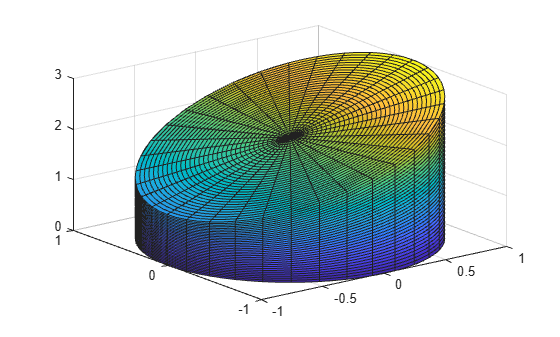### Apply Rotation and Translation to Surface Plot

Apply rotation and translation to the surface plot of a torus.

A torus can be defined parametrically by

$\begin{array}{l}\mathit{x}\left(\theta \text{\hspace{0.17em}},\phi \text{\hspace{0.17em}}\right)=\left(\mathit{R}+\mathit{a}\text{\hspace{0.17em}}\mathrm{cos}\text{\hspace{0.17em}}\theta \right)\text{\hspace{0.17em}}\mathrm{cos}\text{\hspace{0.17em}}\phi \\ \mathit{y}\left(\theta \text{\hspace{0.17em}},\phi \text{\hspace{0.17em}}\right)=\left(\mathit{R}+\mathit{a}\text{\hspace{0.17em}}\mathrm{cos}\text{\hspace{0.17em}}\theta \right)\text{\hspace{0.17em}}\mathrm{sin}\text{\hspace{0.17em}}\phi \\ \mathit{z}\left(\theta \text{\hspace{0.17em}},\phi \text{\hspace{0.17em}}\right)=\mathit{a}\text{\hspace{0.17em}}\mathrm{sin}\text{\hspace{0.17em}}\phi \end{array}$

where

• $\theta \text{\hspace{0.17em}}$ is the polar angle and $\phi \text{\hspace{0.17em}}$ is the azimuthal angle

• $\mathit{a}$ is the radius of the tube

• $\mathit{R}$ is the distance from the center of the tube to the center of the torus

Define the values for $a$ and $R$ as 1 and 5, respectively. Plot the torus using fsurf.

syms theta phi a = 1; R = 4; x = (R + a*cos(theta))*cos(phi); y = (R + a*cos(theta))*sin(phi); z = a*sin(theta); fsurf(x,y,z,[0 2*pi 0 2*pi]) hold on

Apply rotation to the torus around the $x$-axis. Define the rotation matrix. Rotate the torus by 90 degrees or $\pi /2$ radians.

alpha = pi/2; Rx = [1 0 0; 0 cos(alpha) -sin(alpha); 0 sin(alpha) cos(alpha)]; r = [x; y; z]; r_90 = Rx*r;

Shift the center of the torus by 5 along the $x$-axis. Add a second plot of the rotated and translated torus to the existing graph.

fsurf(r_90(1)+5,r_90(2),r_90(3)) axis([-5 10 -5 10 -5 5]) hold off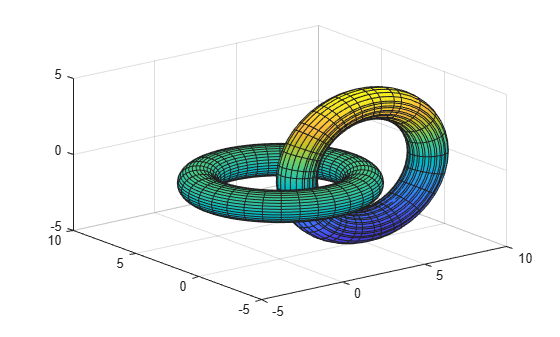## Input Arguments

collapse all

Expression or function to be plotted, specified as a symbolic expression or function.

Plotting interval for x- and y-axes, specified as a vector of two numbers. The default is [-5 5].

Plotting interval for x- and y-axes, specified as a vector of four numbers. The default is [-5 5 -5 5].

Parametric functions of u and v, specified as a symbolic expression or function.

Plotting interval for u and v axes, specified as a vector of two numbers. The default is [-5 5].

Plotting interval for u and v, specified as a vector of four numbers. The default is [-5 5 -5 5].

Axes object. If you do not specify an axes object, then fsurf uses the current axes.

Line style, marker symbol, and color, specified as a character vector. The elements of the character vector can appear in any order, and you can omit one or more options from the character vector specifier.

Example: '--or' is a red surface with circle markers and dashed lines

SpecifierLine Style
-Solid line (default)
--Dashed line
:Dotted line
-.Dash-dot line
SpecifierMarker
oCircle
+Plus sign
*Asterisk
.Point
xCross
sSquare
dDiamond
^Upward-pointing triangle
vDownward-pointing triangle
>Right-pointing triangle
<Left-pointing triangle
pPentagram
hHexagram
SpecifierColor

y

yellow

m

magenta

c

cyan

r

red

g

green

b

blue

w

white

k

black

### Name-Value Pair Arguments

Specify optional comma-separated pairs of Name,Value arguments. Name is the argument name and Value is the corresponding value. Name must appear inside quotes. You can specify several name and value pair arguments in any order as Name1,Value1,...,NameN,ValueN.

Example: 'Marker','o','MarkerFaceColor','red'

The properties listed here are only a subset. For a complete list, see FunctionSurface Properties.

Number of evaluation points per direction, specified as a number. The default is 35. Because fsurf objects use adaptive evaluation, the actual number of evaluation points is greater.

Example: 100

Display contour plot under plot, specified as 'on' or 'off', or as numeric or logical 1 (true) or 0 (false). A value of 'on' is equivalent to true, and 'off' is equivalent to false. Thus, you can use the value of this property as a logical value. The value is stored as an on/off logical value of type matlab.lang.OnOffSwitchState.

Line color, specified as 'interp', an RGB triplet, a hexadecimal color code, a color name, or a short name. The default RGB triplet value of [0 0 0] corresponds to black. The 'interp' value colors the edges based on the ZData values.

For a custom color, specify an RGB triplet or a hexadecimal color code.

• An RGB triplet is a three-element row vector whose elements specify the intensities of the red, green, and blue components of the color. The intensities must be in the range [0,1]; for example, [0.4 0.6 0.7].

• A hexadecimal color code is a character vector or a string scalar that starts with a hash symbol (#) followed by three or six hexadecimal digits, which can range from 0 to F. The values are not case sensitive. Thus, the color codes '#FF8800', '#ff8800', '#F80', and '#f80' are equivalent.

Alternatively, you can specify some common colors by name. This table lists the named color options, the equivalent RGB triplets, and hexadecimal color codes.

Color NameShort NameRGB TripletHexadecimal Color CodeAppearance
'red''r'[1 0 0]'#FF0000''green''g'[0 1 0]'#00FF00''blue''b'[0 0 1]'#0000FF''cyan' 'c'[0 1 1]'#00FFFF''magenta''m'[1 0 1]'#FF00FF''yellow''y'[1 1 0]'#FFFF00''black''k'[0 0 0]'#000000''white''w'[1 1 1]'#FFFFFF''none'Not applicableNot applicableNot applicableNo color

Here are the RGB triplets and hexadecimal color codes for the default colors MATLAB® uses in many types of plots.

[0 0.4470 0.7410]'#0072BD'[0.8500 0.3250 0.0980]'#D95319'[0.9290 0.6940 0.1250]'#EDB120'[0.4940 0.1840 0.5560]'#7E2F8E'[0.4660 0.6740 0.1880]'#77AC30'[0.3010 0.7450 0.9330]'#4DBEEE'[0.6350 0.0780 0.1840]'#A2142F'Line style, specified as one of the options listed in this table.

Line StyleDescriptionResulting Line
'-'Solid line'--'Dashed line':'Dotted line'-.'Dash-dotted line'none'No lineNo line

Line width, specified as a positive value in points, where 1 point = 1/72 of an inch. If the line has markers, then the line width also affects the marker edges.

The line width cannot be thinner than the width of a pixel. If you set the line width to a value that is less than the width of a pixel on your system, the line displays as one pixel wide.

Marker symbol, specified as one of the values in this table. By default, a line does not have markers. Add markers at selected points along the line by specifying a marker.

ValueDescription
'o'Circle
'+'Plus sign
'*'Asterisk
'.'Point
'x'Cross
'square' or 's'Square
'diamond' or 'd'Diamond
'^'Upward-pointing triangle
'v'Downward-pointing triangle
'>'Right-pointing triangle
'<'Left-pointing triangle
'pentagram' or 'p'Five-pointed star (pentagram)
'hexagram' or 'h'Six-pointed star (hexagram)
'none'No markers

Marker outline color, specified as 'auto', an RGB triplet, a hexadecimal color code, a color name, or a short name. The default value of 'auto' uses the same color as the EdgeColor property.

For a custom color, specify an RGB triplet or a hexadecimal color code.

• An RGB triplet is a three-element row vector whose elements specify the intensities of the red, green, and blue components of the color. The intensities must be in the range [0,1]; for example, [0.4 0.6 0.7].

• A hexadecimal color code is a character vector or a string scalar that starts with a hash symbol (#) followed by three or six hexadecimal digits, which can range from 0 to F. The values are not case sensitive. Thus, the color codes '#FF8800', '#ff8800', '#F80', and '#f80' are equivalent.

Alternatively, you can specify some common colors by name. This table lists the named color options, the equivalent RGB triplets, and hexadecimal color codes.

Color NameShort NameRGB TripletHexadecimal Color CodeAppearance
'red''r'[1 0 0]'#FF0000''green''g'[0 1 0]'#00FF00''blue''b'[0 0 1]'#0000FF''cyan' 'c'[0 1 1]'#00FFFF''magenta''m'[1 0 1]'#FF00FF''yellow''y'[1 1 0]'#FFFF00''black''k'[0 0 0]'#000000''white''w'[1 1 1]'#FFFFFF''none'Not applicableNot applicableNot applicableNo color

Here are the RGB triplets and hexadecimal color codes for the default colors MATLAB uses in many types of plots.

[0 0.4470 0.7410]'#0072BD'[0.8500 0.3250 0.0980]'#D95319'[0.9290 0.6940 0.1250]'#EDB120'[0.4940 0.1840 0.5560]'#7E2F8E'[0.4660 0.6740 0.1880]'#77AC30'[0.3010 0.7450 0.9330]'#4DBEEE'[0.6350 0.0780 0.1840]'#A2142F'Example: [0.5 0.5 0.5]

Example: 'blue'

Example: '#D2F9A7'

Marker fill color, specified as 'auto', an RGB triplet, a hexadecimal color code, a color name, or a short name. The 'auto' value uses the same color as the MarkerEdgeColor property.

For a custom color, specify an RGB triplet or a hexadecimal color code.

• An RGB triplet is a three-element row vector whose elements specify the intensities of the red, green, and blue components of the color. The intensities must be in the range [0,1]; for example, [0.4 0.6 0.7].

• A hexadecimal color code is a character vector or a string scalar that starts with a hash symbol (#) followed by three or six hexadecimal digits, which can range from 0 to F. The values are not case sensitive. Thus, the color codes '#FF8800', '#ff8800', '#F80', and '#f80' are equivalent.

Alternatively, you can specify some common colors by name. This table lists the named color options, the equivalent RGB triplets, and hexadecimal color codes.

Color NameShort NameRGB TripletHexadecimal Color CodeAppearance
'red''r'[1 0 0]'#FF0000''green''g'[0 1 0]'#00FF00''blue''b'[0 0 1]'#0000FF''cyan' 'c'[0 1 1]'#00FFFF''magenta''m'[1 0 1]'#FF00FF''yellow''y'[1 1 0]'#FFFF00''black''k'[0 0 0]'#000000''white''w'[1 1 1]'#FFFFFF''none'Not applicableNot applicableNot applicableNo color

Here are the RGB triplets and hexadecimal color codes for the default colors MATLAB uses in many types of plots.

[0 0.4470 0.7410]'#0072BD'[0.8500 0.3250 0.0980]'#D95319'[0.9290 0.6940 0.1250]'#EDB120'[0.4940 0.1840 0.5560]'#7E2F8E'[0.4660 0.6740 0.1880]'#77AC30'[0.3010 0.7450 0.9330]'#4DBEEE'[0.6350 0.0780 0.1840]'#A2142F'Example: [0.3 0.2 0.1]

Example: 'green'

Example: '#D2F9A7'

Marker size, specified as a positive value in points, where 1 point = 1/72 of an inch.

## Output Arguments

collapse all

One or more objects, returned as a scalar or a vector. The object is either a function surface object or parameterized surface object, depending on the type of plot. You can use these objects to query and modify properties of a specific line. For details, see FunctionSurface Properties and ParameterizedFunctionSurface Properties.

## Algorithms

fsurf assigns the symbolic variables in f to the x axis, then the y axis, and symvar determines the order of the variables to be assigned. Therefore, variable and axis names might not correspond. To force fsurf to assign x or y to its corresponding axis, create the symbolic function to plot, then pass the symbolic function to fsurf.

For example, the following code plots f(x,y) = sin(y) in two ways. The first way forces the waves to oscillate with respect to the y axis. The second way assigns y to the x axis because it is the first (and only) variable in the symbolic function.

syms x y; f(x,y) = sin(y); figure; subplot(2,1,1) fsurf(f); subplot(2,1,2) fsurf(f(x,y)); % Or fsurf(sin(y));### Topics

#### Mathematical Modeling with Symbolic Math Toolbox

Get examples and videos